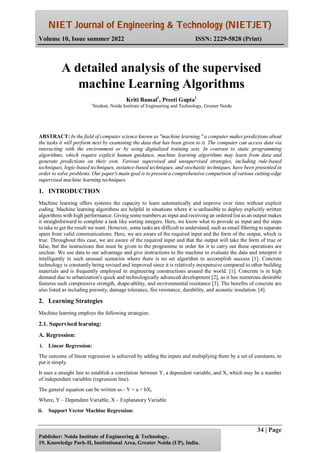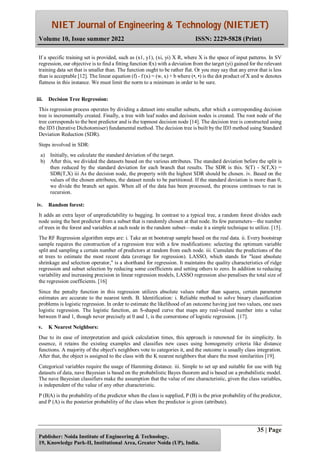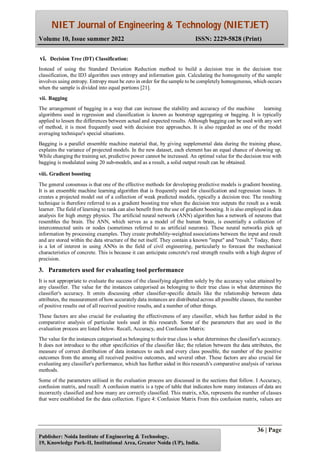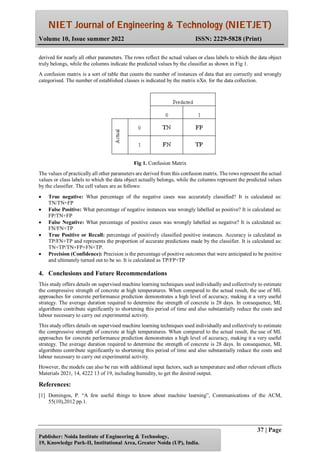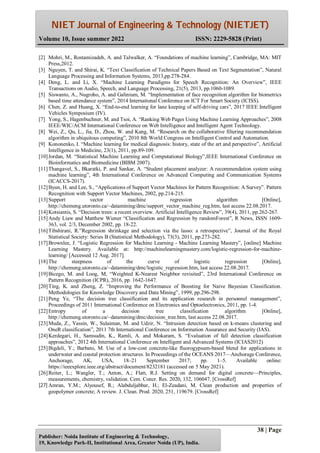Prochain SlideShare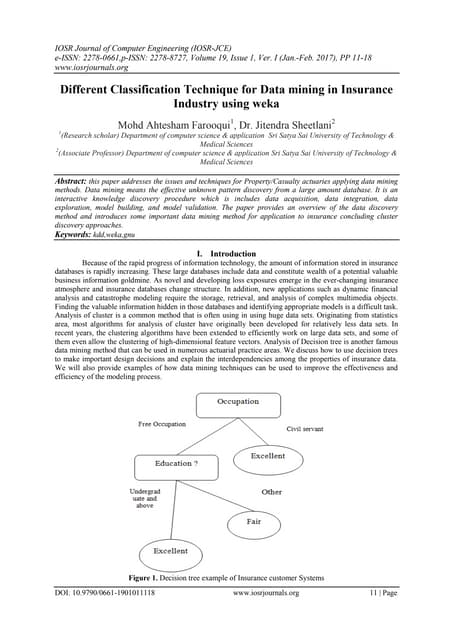Different Classification Technique for Data mining in Insurance Industry usin...
Chargement dans ... 3
1 sur 5

## RecommandéDifferent Classification Technique for Data mining in Insurance Industry usin...IOSRjournaljceIRJET- A Detailed Study on Classification Techniques for Data MiningIRJET JournalEstimating project development effort using clustered regression approachcsanditESTIMATING PROJECT DEVELOPMENT EFFORT USING CLUSTERED REGRESSION APPROACHcscpconfIRJET- Supervised Learning Classification Algorithms ComparisonIRJET JournalIRJET- Supervised Learning Classification Algorithms ComparisonIRJET Journal

### A detailed analysis of the supervised machine Learning Algorithms

1. NIET Journal of Engineering & Technology (NIETJET) Volume 10, Issue summer 2022 ISSN: 2229-5828 (Print) 34 | Page Publisher: Noida Institute of Engineering & Technology, 19, Knowledge Park-II, Institutional Area, Greater Noida (UP), India. ABSTRACT: In the field of computer science known as "machine learning," a computer makes predictions about the tasks it will perform next by examining the data that has been given to it. The computer can access data via interacting with the environment or by using digitalized training sets. In contrast to static programming algorithms, which require explicit human guidance, machine learning algorithms may learn from data and generate predictions on their own. Various supervised and unsupervised strategies, including rule-based techniques, logic-based techniques, instance-based techniques, and stochastic techniques, have been presented in order to solve problems. Our paper's main goal is to present a comprehensive comparison of various cutting-edge supervised machine learning techniques. 1. INTRODUCTION Machine learning offers systems the capacity to learn automatically and improve over time without explicit coding. Machine learning algorithms are helpful in situations where it is unfeasible to deploy explicitly written algorithms with high performance. Giving some numbers as input and receiving an ordered list as an output makes it straightforward to complete a task like sorting integers. Here, we know what to provide as input and the steps to take to get the result we want. However, some tasks are difficult to understand, such as email filtering to separate spam from valid communications. Here, we are aware of the required input and the form of the output, which is true. Throughout this case, we are aware of the required input and that the output will take the form of true or false, but the instructions that must be given to the programme in order for it to carry out these operations are unclear. We use data to our advantage and give instructions to the machine to evaluate the data and interpret it intelligently in such unusual scenarios where there is no set algorithm to accomplish success . Concrete technology is constantly being revised and improved since it is relatively inexpensive compared to other building materials and is frequently employed in engineering constructions around the world. . Concrete is in high demand due to urbanization's quick and technologically advanced development , as it has numerous desirable features such compressive strength, shape-ability, and environmental resistance . The benefits of concrete are also listed as including porosity, damage tolerance, fire resistance, durability, and acoustic insulation. . 2. Learning Strategies Machine learning employs the following strategies: 2.1. Supervised learning: A. Regression: i. Linear Regression: The outcome of linear regression is achieved by adding the inputs and multiplying them by a set of constants, to put it simply. It uses a straight line to establish a correlation between Y, a dependent variable, and X, which may be a number of independent variables (regression line). The general equation can be written as - Y = a + bX, Where, Y – Dependent Variable, X – Explanatory Variable ii. Support Vector Machine Regression: A detailed analysis of the supervised machine Learning Algorithms Kriti Bansal1 , Preeti Gupta1 1 Student, Noida Institute of Engineering and Technology, Greater Noida
2. NIET Journal of Engineering & Technology (NIETJET) Volume 10, Issue summer 2022 ISSN: 2229-5828 (Print) 35 | Page Publisher: Noida Institute of Engineering & Technology, 19, Knowledge Park-II, Institutional Area, Greater Noida (UP), India. If a specific training set is provided, such as (x1, y1), (xi, yi) X R, where X is the space of input patterns. In SV regression, our objective is to find a fitting function f(x) with a deviation from the target (yi) gained for the relevant training data set that is smaller than. The function ought to be rather flat. Or you may say that any error that is less than is acceptable . The linear equation (f) - f (x) = (w, x) + b where (•, •) is the dot product of X and w denotes flatness in this instance. We must limit the norm to a minimum in order to be sure. iii. Decision Tree Regression: This regression process operates by dividing a dataset into smaller subsets, after which a corresponding decision tree is incrementally created. Finally, a tree with leaf nodes and decision nodes is created. The root node of the tree corresponds to the best predictor and is the topmost decision node . The decision tree is constructed using the ID3 (Iterative Dichotomiser) fundamental method. The decision tree is built by the ID3 method using Standard Deviation Reduction (SDR). Steps involved in SDR: a) Initially, we calculate the standard deviation of the target. b) After this, we divided the datasets based on the various attributes. The standard deviation before the split is then reduced by the standard deviation for each branch that results. The SDR is this. S(T) - S(T,X) = SDR(T,X) iii As the decision node, the property with the highest SDR should be chosen. iv. Based on the values of the chosen attributes, the dataset needs to be partitioned. If the standard deviation is more than 0, we divide the branch set again. When all of the data has been processed, the process continues to run in recursion. iv. Random forest: It adds an extra layer of unpredictability to bagging. In contrast to a typical tree, a random forest divides each node using the best predictor from a subset that is randomly chosen at that node. Its few parameters—the number of trees in the forest and variables at each node in the random subset—make it a simple technique to utilize. . The RF Regression algorithm steps are: i. Take an nt bootstrap sample based on the real data. ii. Every bootstrap sample requires the construction of a regression tree with a few modifications: selecting the optimum variable split and sampling a certain number of predictors at random from each node. iii. Cumulate the predictions of the nt trees to estimate the most recent data (average for regression). LASSO, which stands for "least absolute shrinkage and selection operator," is a shorthand for regression. It maintains the quality characteristics of ridge regression and subset selection by reducing some coefficients and setting others to zero. In addition to reducing variability and increasing precision in linear regression models, LASSO regression also penalises the total size of the regression coefficients.  Since the penalty function in this regression utilizes absolute values rather than squares, certain parameter estimates are accurate to the nearest tenth. B. Identification: i. Reliable method to solve binary classification problems is logistic regression. In order to estimate the likelihood of an outcome having just two values, one uses logistic regression. The logistic function, an S-shaped curve that maps any real-valued number into a value between 0 and 1, though never precisely at 0 and 1, is the cornerstone of logistic regression. . v. K Nearest Neighbors: Due to its ease of interpretation and quick calculation times, this approach is renowned for its simplicity. In essence, it retains the existing examples and classifies new cases using homogeneity criteria like distance functions. A majority of the object's neighbors vote to categories it, and the outcome is usually class integration. After that, the object is assigned to the class with the K nearest neighbors that share the most similarities . Categorical variables require the usage of Hamming distance. iii. Simple to set up and suitable for use with big datasets of data, nave Bayesian is based on the probabilistic Bayes theorem and is based on a probabilistic model. The nave Bayesian classifiers make the assumption that the value of one characteristic, given the class variables, is independent of the value of any other characteristic. P (B|A) is the probability of the predictor when the class is supplied, P (B) is the prior probability of the predictor, and P (A) is the posterior probability of the class when the predictor is given (attribute).
3. NIET Journal of Engineering & Technology (NIETJET) Volume 10, Issue summer 2022 ISSN: 2229-5828 (Print) 36 | Page Publisher: Noida Institute of Engineering & Technology, 19, Knowledge Park-II, Institutional Area, Greater Noida (UP), India. vi. Decision Tree (DT) Classification: Instead of using the Standard Deviation Reduction method to build a decision tree in the decision tree classification, the ID3 algorithm uses entropy and information gain. Calculating the homogeneity of the sample involves using entropy. Entropy must be zero in order for the sample to be completely homogeneous, which occurs when the sample is divided into equal portions . vii. Bagging The arrangement of bagging in a way that can increase the stability and accuracy of the machine learning algorithms used in regression and classification is known as bootstrap aggregating or bagging. It is typically applied to lessen the differences between actual and expected results. Although bagging can be used with any sort of method, it is most frequently used with decision tree approaches. It is also regarded as one of the model averaging technique's special situations. Bagging is a parallel ensemble machine material that, by giving supplemental data during the training phase, explains the variance of projected models. In the new dataset, each element has an equal chance of showing up. While changing the training set, predictive power cannot be increased. An optimal value for the decision tree with bagging is modulated using 20 sub-models, and as a result, a solid output result can be obtained. viii. Gradient boosting The general consensus is that one of the effective methods for developing predictive models is gradient boosting. It is an ensemble machine learning algorithm that is frequently used for classification and regression issues. It creates a projected model out of a collection of weak predicted models, typically a decision tree. The resulting technique is therefore referred to as a gradient boosting tree when the decision tree outputs the result as a weak learner. The field of learning to rank can also benefit from the use of gradient boosting. It is also employed in data analysis for high energy physics. The artificial neural network (ANN) algorithm has a network of neurons that resembles the brain. The ANN, which serves as a model of the human brain, is essentially a collection of interconnected units or nodes (sometimes referred to as artificial neurons). These neural networks pick up information by processing examples. They create probability-weighted associations between the input and result and are stored within the data structure of the net itself. They contain a known "input" and "result." Today, there is a lot of interest in using ANNs in the field of civil engineering, particularly to forecast the mechanical characteristics of concrete. This is because it can anticipate concrete's real strength results with a high degree of precision. 3. Parameters used for evaluating tool performance It is not appropriate to evaluate the success of the classifying algorithm solely by the accuracy value attained by any classifier. The value for the instances categorised as belonging to their true class is what determines the classifier's accuracy. It omits discussing other classifier-specific details like the relationship between data attributes, the measurement of how accurately data instances are distributed across all possible classes, the number of positive results out of all received positive results, and a number of other things. These factors are also crucial for evaluating the effectiveness of any classifier, which has further aided in the comparative analysis of particular tools used in this research. Some of the parameters that are used in the evaluation process are listed below. Recall, Accuracy, and Confusion Matrix: The value for the instances categorised as belonging to their true class is what determines the classifier's accuracy. It does not introduce to the other specificities of the classifier like; the relation between the data attributes, the measure of correct distribution of data instances to each and every class possible, the number of the positive outcomes from the among all received positive outcomes, and several other. These factors are also crucial for evaluating any classifier's performance, which has further aided in this research's comparative analysis of various methods. Some of the parameters utilised in the evaluation process are discussed in the sections that follow. I Accuracy, confusion matrix, and recall: A confusion matrix is a type of table that indicates how many instances of data are incorrectly classified and how many are correctly classified. This matrix, nXn, represents the number of classes that were established for the data collection. Figure 4: Confusion Matrix From this confusion matrix, values are
4. NIET Journal of Engineering & Technology (NIETJET) Volume 10, Issue summer 2022 ISSN: 2229-5828 (Print) 37 | Page Publisher: Noida Institute of Engineering & Technology, 19, Knowledge Park-II, Institutional Area, Greater Noida (UP), India. derived for nearly all other parameters. The rows reflect the actual values or class labels to which the data object truly belongs, while the columns indicate the predicted values by the classifier as shown in Fig 1. A confusion matrix is a sort of table that counts the number of instances of data that are correctly and wrongly categorised. The number of established classes is indicated by the matrix nXn. for the data collection. Fig 1. Confusion Matrix The values of practically all other parameters are derived from this confusion matrix. The rows represent the actual values or class labels to which the data object actually belongs, while the columns represent the predicted values by the classifier. The cell values are as follows:  True negative: What percentage of the negative cases was accurately classified? It is calculated as: TN/TN+FP  False Positive: What percentage of negative instances was wrongly labelled as positive? It is calculated as: FP/TN+FP  False Negative: What percentage of positive cases was wrongly labelled as negative? It is calculated as: FN/FN+TP  True Positive or Recall: percentage of positively classified positive instances. Accuracy is calculated as TP/FN+TP and represents the proportion of accurate predictions made by the classifier. It is calculated as: TN+TP/TN+FP+FN+TP.  Precision (Confidence): Precision is the percentage of positive outcomes that were anticipated to be positive and ultimately turned out to be so. It is calculated as TP/FP+TP 4. Conclusions and Future Recommendations This study offers details on supervised machine learning techniques used individually and collectively to estimate the compressive strength of concrete at high temperatures. When compared to the actual result, the use of ML approaches for concrete performance prediction demonstrates a high level of accuracy, making it a very useful strategy. The average duration required to determine the strength of concrete is 28 days. In consequence, ML algorithms contribute significantly to shortening this period of time and also substantially reduce the costs and labour necessary to carry out experimental activity. This study offers details on supervised machine learning techniques used individually and collectively to estimate the compressive strength of concrete at high temperatures. When compared to the actual result, the use of ML approaches for concrete performance prediction demonstrates a high level of accuracy, making it a very useful strategy. The average duration required to determine the strength of concrete is 28 days. In consequence, ML algorithms contribute significantly to shortening this period of time and also substantially reduce the costs and labour necessary to carry out experimental activity. However, the models can also be run with additional input factors, such as temperature and other relevant effects Materials 2021, 14, 4222 13 of 19, including humidity, to get the desired output. References:  Domingos, P. “A few useful things to know about machine learning”, Communications of the ACM, 55(10),2012 pp.1.
5. NIET Journal of Engineering & Technology (NIETJET) Volume 10, Issue summer 2022 ISSN: 2229-5828 (Print) 38 | Page Publisher: Noida Institute of Engineering & Technology, 19, Knowledge Park-II, Institutional Area, Greater Noida (UP), India.  Mohri, M., Rostamizadeh, A. and Talwalker, A. “Foundations of machine learning”, Cambridge, MA: MIT Press,2012.  Nguyen, T. and Shirai, K. “Text Classification of Technical Papers Based on Text Segmentation”, Natural Language Processing and Information Systems, 2013,pp.278-284.  Deng, L. and Li, X. “Machine Learning Paradigms for Speech Recognition: An Overview”, IEEE Transactions on Audio, Speech, and Language Processing, 21(5), 2013, pp.1060-1089.  Siswanto, A., Nugroho, A. and Galinium, M. “Implementation of face recognition algorithm for biometrics based time attendance system”, 2014 International Conference on ICT For Smart Society (ICISS).  Chen, Z. and Huang, X. “End-to-end learning for lane keeping of self-driving cars”, 2017 IEEE Intelligent Vehicles Symposium (IV).  Yong, S., Hagenbuchner, M. and Tsoi, A. “Ranking Web Pages Using Machine Learning Approaches”, 2008 IEEE/WIC/ACM International Conference on Web Intelligence and Intelligent Agent Technology.  Wei, Z., Qu, L., Jia, D., Zhou, W. and Kang, M. “Research on the collaborative filtering recommendation algorithm in ubiquitous computing”, 2010 8th World Congress on Intelligent Control and Automation.  Kononenko, I. “Machine learning for medical diagnosis: history, state of the art and perspective”, Artificial Intelligence in Medicine, 23(1), 2011, pp.89-109. Jordan, M. “Statistical Machine Learning and Computational Biology”,IEEE International Conference on Bioinformatics and Biomedicine (BIBM 2007). Thangavel, S., Bkaratki, P. and Sankar, A. “Student placement analyzer: A recommendation system using machine learning”, 4th International Conference on Advanced Computing and Communication Systems (ICACCS-2017). Byun, H. and Lee, S., “Applications of Support Vector Machines for Pattern Recognition: A Survey”. Pattern Recognition with Support Vector Machines, 2002, pp.214-215. Support vector machine regression algorithm [Online], http://chemeng.utoronto.ca/~datamining/dmc/support_vector_machine_reg.htm, last access 22.08.2017. Kotsiantis, S. “Decision trees: a recent overview. Artificial Intelligence Review”, 39(4), 2011, pp.262-267. Andy Liaw and Matthew Wiener “Classification and Regression by randomForest”, R News, ISSN 1609- 363, vol. 2/3, December 2002, pp. 18-22. Tibshirani, R.”Regression shrinkage and selection via the lasso: a retrospective”, Journal of the Royal Statistical Society: Series B (Statistical Methodology), 73(3), 2011, pp.273-282. Brownlee, J. “Logistic Regression for Machine Learning - Machine Learning Mastery”, [online] Machine Learning Mastery. Available at: http://machinelearningmastery.com/logistic-regression-for-machine- learning/ [Accessed 12 Aug. 2017]. The steepness of the curve of logistic regression [Online], http://chemeng.utoronto.ca/~datamining/dmc/logistic_regression.htm, last access 22.08.2017. Bicego, M. and Loog, M, “Weighted K-Nearest Neighbor revisited”, 23rd International Conference on Pattern Recognition (ICPR), 2016, pp. 1642-1647. Ting, K. and Zheng, Z. “Improving the Performance of Boosting for Naive Bayesian Classification. Methodologies for Knowledge Discovery and Data Mining”, 1999, pp.296-298. Peng Ye, “The decision tree classification and its application research in personnel management”, Proceedings of 2011 International Conference on Electronics and Optoelectronics, 2011, pp. 1-4. Entropy of a decision tree classification algorithm [Online], http://chemeng.utoronto.ca/~datamining/dmc/decision_tree.htm, last access 22.08.2017. Muda, Z., Yassin, W., Sulaiman, M. and Udzir, N. “Intrusion detection based on k-means clustering and OneR classification”, 2011 7th International Conference on Information Assurance and Security (IAS). Kerdegari, H., Samsudin, K., Ramli, A. and Mokaram, S. “Evaluation of fall detection classification approaches”, 2012 4th International Conference on Intelligent and Advanced Systems (ICIAS2012) Bigdeli, Y.; Barbato, M. Use of a low-cost concrete-like fluorogypsum-based blend for applications in underwater and coastal protection structures. In Proceedings of the OCEANS 2017—Anchorage Conference, Anchorage, AK, USA, 18–21 September 2017; pp. 1–5. Available online: https://ieeexplore.ieee.org/abstract/document/8232181 (accessed on 5 May 2021). Reiter, L.; Wangler, T.; Anton, A.; Flatt, R.J. Setting on demand for digital concrete—Principles, measurements, chemistry, validation. Cem. Concr. Res. 2020, 132, 106047. [CrossRef]  Amran, Y.M.; Alyousef, R.; Alabduljabbar, H.; El-Zeadani, M. Clean production and properties of geopolymer concrete; A review. J. Clean. Prod. 2020, 251, 119679. [CrossRef]